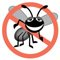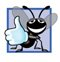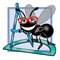# Another Java Application: Adding Integers

### Another Java Application Adding Integers

Our next application reads (or inputs) two integers (whole numbers, like 22, 7, 0 and 1024) typed by a user at the keyboard, computes the sum of the values and displays the result. This program must keep track of the numbers supplied by the user for the calculation later in the program. Programs remember numbers and other data in the computer's memory and access that data through program elements called variables. The program of Fig. 2.7 demonstrates these concepts. In the sample output, we use highlighting to differentiate between the user's input and the program's output.

Figure 2.7. Addition program that displays the sum of two numbers.

``` 1 // Fig. 2.7: Addition.java
2 // Addition program that displays the sum of two numbers.
3 import java.util.Scanner; // program uses class Scanner
4
6 {
7 // main method begins execution of Java application
8 public static void main( String args[] )
9 {
10 // create Scanner to obtain input from command window
11 Scanner input = new Scanner( System.in );
12
13 int number1; // first number to add
14 int number2; // second number to add
15 int sum; // sum of number1 and number2
16
17 System.out.print( "Enter first integer: " ); // prompt
18 number1 = input.nextInt(); // read first number from user
19
20 System.out.print( "Enter second integer: " ); // prompt
21 number2 = input.nextInt(); // read second number from user
22
23 sum = number1 + number2; // add numbers
24
25 System.out.printf( "Sum is %d
", sum ); // display sum
26
27 } // end method main
28
29 } // end class Addition
```

 ```Enter first integer: 45 Enter second integer: 72 Sum is 117 ```

Lines 12

``` // Fig. 2.7: Addition.java
// Addition program that displays the sum of two numbers.
```

state the figure number, file name and purpose of the program.

Line 3

``` import java.util.Scanner; // program uses class Scanner
```

is an import declaration that helps the compiler locate a class that is used in this program. A great strength of Java is its rich set of predefined classes that programmers can reuse rather than "reinventing the wheel." These classes are grouped into packagesnamed collections of classes. Collectively, Java's packages are referred to as the Java class library, or the Java Application Programming Interface (Java API). Programmers use import declarations to identify the predefined classes used in a Java program. The import declaration in line 3 indicates that this example uses Java's predefined Scanner class (discussed shortly) from package java.util. Then the compiler attempts to ensure that you use class Scanner correctly.

Common Programming Error 2.8All import declarations must appear before the first class declaration in the file. Placing an import declaration inside a class declaration's body or after a class declaration is a syntax error.

Error-Prevention Tip 2.7Forgetting to include an import declaration for a class used in your program typically results in a compilation error containing a message such as "cannot resolve symbol." When this occurs, check that you provided the proper import declarations and that the names in the import declarations are spelled correctly, including proper use of uppercase and lowercase letters.

Line 5

``` public class Addition
```

begins the declaration of class Addition. The file name for this public class must be Addition.java. Remember that the body of each class declaration starts with an opening left brace (line 6), {, and ends with a closing right brace (line 29), }.

The application begins execution with method main (lines 827). The left brace (line 9) marks the beginning of main's body, and the corresponding right brace (line 27) marks the end of main's body. Note that method main is indented one level in the body of class Addition and that the code in the body of main is indented another level for readability.

Line 11

``` Scanner input = new Scanner( System.in);
```

is a variable declaration statement (also called a declaration) that specifies the name and type of a variable (input) that is used in this program. A variable is a location in the computer's memory where a value can be stored for use later in a program. All variables must be declared with a name and a type before they can be used. A variable's name enables the program to access the value of the variable in memory. A variable's name can be any valid identifier. (See Section 2.2 for identifier naming requirements.) A variable's type specifies what kind of information is stored at that location in memory. Like other statements, declaration statements end with a semicolon (;).

The declaration in line 11 specifies that the variable named input is of type Scanner. A Scanner enables a program to read data (e.g., numbers) for use in a program. The data can come from many sources, such as a file on disk or the user at the keyboard. Before using a Scanner, the program must create it and specify the source of the data.

The equal sign (=) in line 11 indicates that Scanner variable input should be initialized (i.e., prepared for use in the program) in its declaration with the result of the expression new Scanner(System.in) to the right of the equal sign. This expression creates a Scanner object that reads data typed by the user at the keyboard. Recall that the standard output object, System.out, allows Java applications to display characters in the command window. Similarly, the standard input object, System.in, enables Java applications to read information typed by the user. So, line 11 creates a Scanner that enables the application to read information typed by the user at the keyboard.

The variable declaration statements at lines 1315

``` int number1; // first number to add
int number2; // second number to add
int sum; // sum of number1 and number2
```

declare that variables number1, number2 and sum are data of type intthese variables will hold integer values (whole numbers such as 7, 11, 0 and 31,914). These variables are not yet initialized. The range of values for an int is 2,147,483,648 to +2,147,483,647. We will soon discuss types float and double, for specifying real numbers, and type char, for specifying character data. Real numbers are numbers that contain decimal points, such as 3.4, 0.0 and 11.19. Variables of type char represent individual characters, such as an uppercase letter (e.g., A), a digit (e.g., 7), a special character (e.g., * or %) or an escape sequence (e.g., the newline character, ). Types such as int, float, double and char are often called primitive types or built-in types. Primitive-type names are keywords and therefore must appear in all lowercase letters. Appendix D summarizes the characteristics of the eight primitive types (boolean, byte, char, short, int, long, float and double).

Variable declaration statements can be split over several lines, with the variable names separated by commas (i.e., a comma-separated list of variable names). Several variables of the same type may be declared in one declaration or in multiple declarations. For example, lines 1315 can also be written as follows:

``` int number1, // first number to add
number2, // second number to add
sum; // sum of number1 and number2
```

Note that we used end-of-line comments in lines 1315. This use of comments is a common programming practice for indicating the purpose of each variable in the program.

Good Programming Practice 2.10Declare each variable on a separate line. This format allows a descriptive comment to be easily inserted next to each declaration.

Good Programming Practice 2.11Choosing meaningful variable names helps a program to be self-documenting (i.e., one can understand the program simply by reading it rather than by reading manuals or viewing an excessive number of comments).

Good Programming Practice 2.12By convention, variable-name identifiers begin with a lowercase letter, and every word in the name after the first word begins with a capital letter. For example, variable-name identifier firstNumber has a capital N in its second word, Number.

Line 17

``` System.out.print( "Enter first integer: " ); // prompt
```

uses System.out.print to display the message "Enter first integer:". This message is called a prompt because it directs the user to take a specific action. Recall from Section 2.2 that identifiers starting with capital letters represent class names. So, System is a class. Class System is part of package java.lang. Notice that class System is not imported with an import declaration at the beginning of the program.

Software Engineering Observation 2.1By default, package java.lang is imported in every Java program; thus, java.lang is the only package in the Java API that does not require an import declaration.

Line 18

` number1 = input.nextInt(); // read first number from user`

uses Scanner object input's nextInt method to obtain an integer from the user at the keyboard. At this point the program waits for the user to type the number and press the Enter key to submit the number to the program.

Technically, the user can type anything as the input value. Our program assumes that the user enters a valid integer value as requested. In this program, if the user types a non-integer value, a runtime logic error will occur and the program will terminate. Chapter 13, Exception Handling, discusses how to make your programs more robust by enabling them to handle such errors. This is also known as making your program fault tolerant.

In line 17, the result of the call to method nextInt (an int value) is placed in variable number1 by using the assignment operator, =. The statement is read as "number1 gets the value of input.nextInt()." Operator = is called a binary operator because it has two operandsnumber1 and the result of the method call input.nextInt(). This statement is called an assignment statement because it is a statement that assigns a value to a variable. Everything to the right of the assignment operator, =, is always evaluated before the assignment is performed.

Good Programming Practice 2.13Place spaces on either side of a binary operator to make it stand out and make the program more readable.

Line 20

``` System.out.print( "Enter second integer: " ); // prompt
```

prompts the user to input the second integer. Line 21

``` number2 = input.nextInt(); // read second number from user
```

reads the second integer and assigns it to variable number2.

Line 23

` sum = number1 + number2; // add numbers`

is an assignment statement that calculates the sum of the variables number1 and number2 and assigns the result to variable sum by using the assignment operator, =. The statement is read as "sum gets the value of number1 + number2." Most calculations are performed in assignment statements. When the program encounters the addition operation, it uses the values stored in the variables number1 and number2 to perform the calculation. In the preceding statement, the addition operator is a binary operatorits two operands are number1 and number2. Portions of statements that contain calculations are called expressions. In fact, an expression is any portion of a statement that has a value associated with it. For example, the value of the expression number1 + number2 is the sum of the numbers. Similarly, the value of the expression input.nextInt() is an integer typed by the user.

After the calculation has been performed, line 25

``` System.out.printf( "Sum is %d
", sum ); // display sum```

uses method System.out.printf to display the sum. The format specifier %d is a place-holder for an int value (in this case the value of sum)the letter d stands for "decimal integer." Note that other than the %d format specifier, the remaining characters in the format string are all fixed text. So method printf displays "Sum is", followed by the value of sum (in the position of the %d format specifier) and a newline.

Note that calculations can also be performed inside printf statements. We could have combined the statements at lines 23 and 25 into the statement

``` System.out.printf( "Sum is %d
", ( number1 + number2 ) );
```

The parentheses around the expression number1 + number2 are not requiredthey are included to emphasize that the value of the expression is output in the position of the %d format specifier.

Java API Documentation

For each new Java API class we use, we indicate the package in which it is located. This package information is important because it helps you locate descriptions of each package and class in the Java API documentation. A Web-based version of this documentation can be found at

java.sun.com/j2se/5.0/docs/api/

The download is approximately 40 megabytes (MB) in size. Appendix G provides an overview of using the Java API documentation.Java How to Program (6th Edition) (How to Program (Deitel))
ISBN: 0131483986
EAN: 2147483647
Year: 2003
Pages: 615